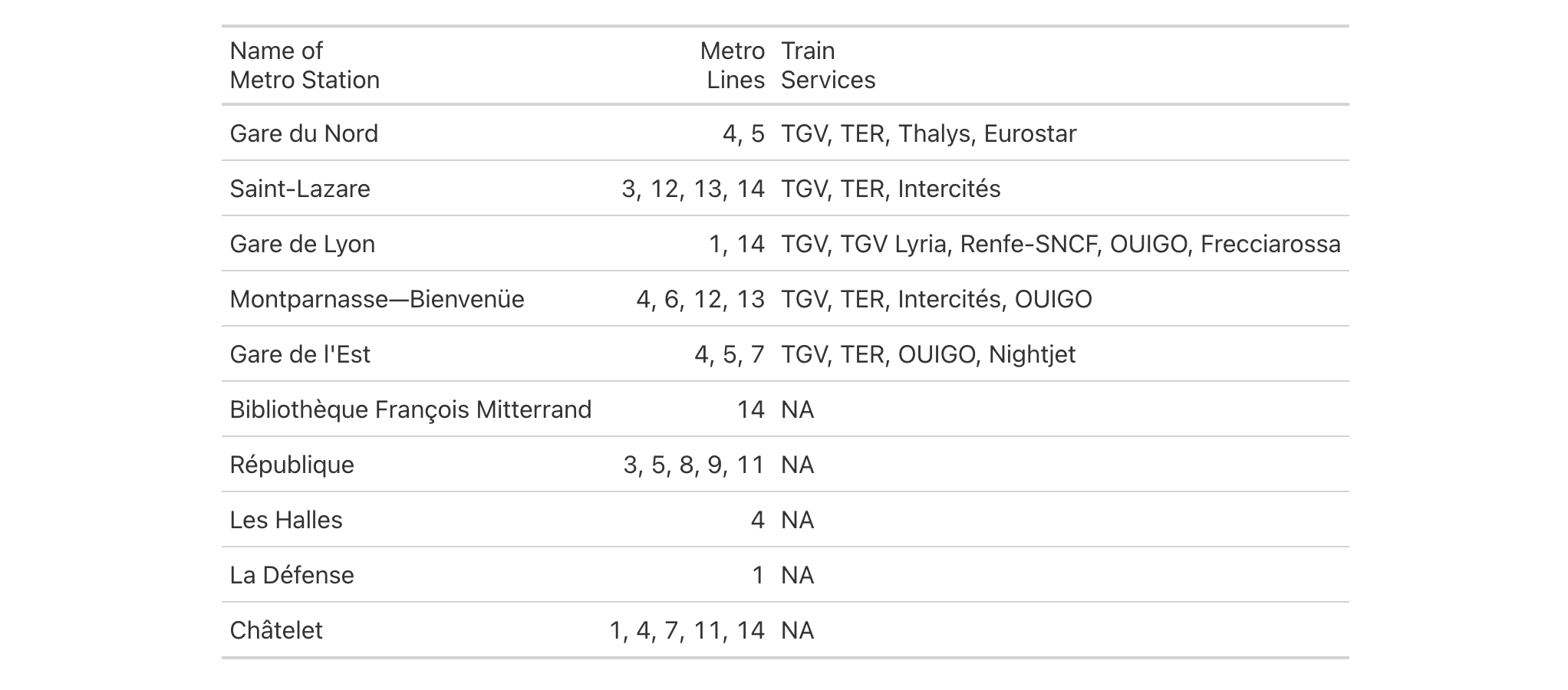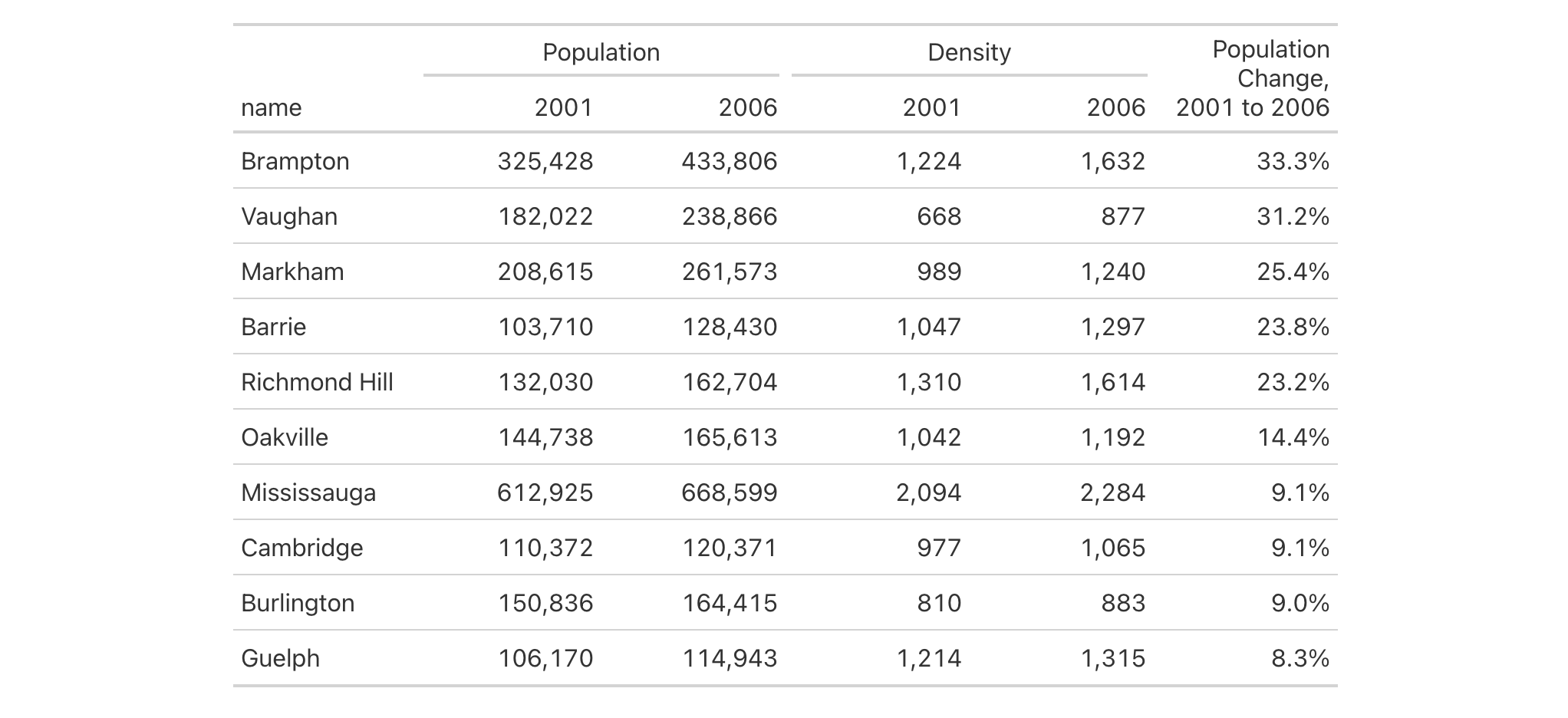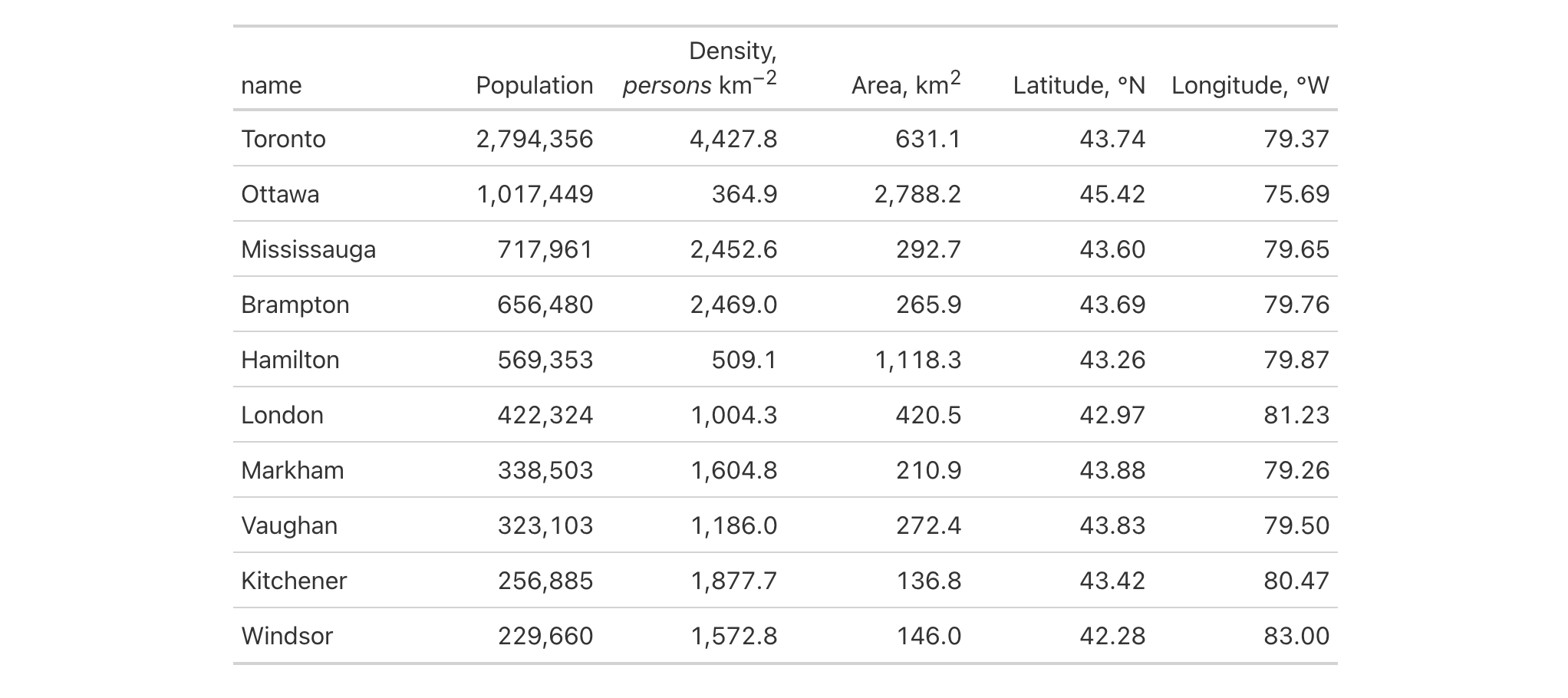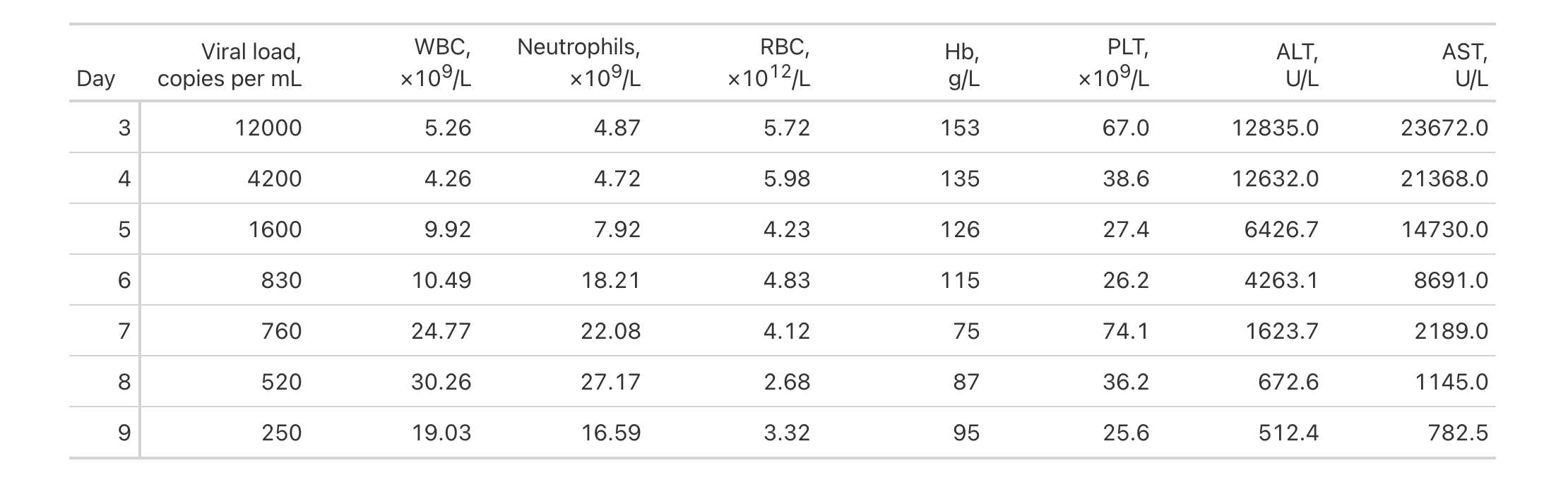# cols_label: Relabel one or more columns In gt: Easily Create Presentation-Ready Display Tables

 cols_label R Documentation

## Relabel one or more columns

### Description

Column labels can be modified from their default values (the names of the columns from the input table data). When you create a gt table object using `gt()`, column names effectively become the column labels. While this serves as a good first approximation, column names as label defaults aren't often as appealing in a gt table as the option for custom column labels. The `cols_label()` function provides the flexibility to relabel one or more columns and we even have the option to use the `md()` or `html()` helper functions for rendering column labels from Markdown or using HTML.

### Usage

``````cols_label(.data, ..., .list = list2(...), .fn = NULL, .process_units = NULL)
``````

### Arguments

 `.data` The gt table data object `⁠obj:⁠` // required This is the gt table object that is commonly created through use of the `gt()` function. `...` Column label assignments `⁠⁠` // required (or, use `.list`) Expressions for the assignment of column labels for the table columns in `.data`. Two-sided formulas (e.g., `⁠ ~ ⁠`) can be used, where the left-hand side corresponds to selections of columns and the right-hand side evaluates to single-length values for the label to apply. Column names should be enclosed in `c()`. Select helpers like `starts_with()`, `ends_with()`, `contains()`, `matches()`, `one_of()`, and `everything()` can be used in the LHS. Named arguments are also valid as input for simple mappings of column name to label text; they should be of the form `⁠ =

### Value

An object of class `gt_tbl`.

### A note on column names and column labels

It's important to note that while columns can be freely relabeled, we continue to refer to columns by their original column names. Column names in a tibble or data frame must be unique whereas column labels in gt have no requirement for uniqueness (which is useful for labeling columns as, say, measurement units that may be repeated several times—usually under different spanner labels). Thus, we can still easily distinguish between columns in other gt function calls (e.g., in all of the `⁠fmt*()⁠` functions) even though we may lose distinguishability between column labels once they have undergone relabeling.

### Incorporating units with gt's units notation

Measurement units are often seen as part of column labels and indeed it can be much more straightforward to include them here rather than using other devices to make readers aware of units for specific columns. The gt package offers the function `cols_units()` to apply units to various columns with an interface that's similar to that of this function. However, it is also possible to define units here along with the column label, obviating the need for pattern syntax that joins the two text components. To do this, we have to surround the portion of text in the label that corresponds to the units definition with `"{{"`/`"}}"`.

Now that we know how to mark text for units definition, we know need to know how to write proper units with the notation. Such notation uses a succinct method of writing units and it should feel somewhat familiar though it is particular to the task at hand. Each unit is treated as a separate entity (parentheses and other symbols included) and the addition of subscript text and exponents is flexible and relatively easy to formulate. This is all best shown with a few examples:

• `"m/s"` and `"m / s"` both render as `"m/s"`

• `"m s^-1"` will appear with the `"-1"` exponent intact

• `"m /s"` gives the the same result, as `"/<unit>"` is equivalent to `"<unit>^-1"`

• `"E_h"` will render an `"E"` with the `"h"` subscript

• `"t_i^2.5"` provides a `t` with an `"i"` subscript and a `"2.5"` exponent

• `"m[_0^2]"` will use overstriking to set both scripts vertically

• `"g/L %C6H12O6%"` uses a chemical formula (enclosed in a pair of `"%"` characters) as a unit partial, and the formula will render correctly with subscripted numbers

• Common units that are difficult to write using ASCII text may be implicitly converted to the correct characters (e.g., the `"u"` in `"ug"`, `"um"`, `"uL"`, and `"umol"` will be converted to the Greek mu symbol; `"degC"` and `"degF"` will render a degree sign before the temperature unit)

• We can transform shorthand symbol/unit names enclosed in `":"` (e.g., `":angstrom:"`, `":ohm:"`, etc.) into proper symbols

• Greek letters can added by enclosing the letter name in `":"`; you can use lowercase letters (e.g., `":beta:"`, `":sigma:"`, etc.) and uppercase letters too (e.g., `":Alpha:"`, `":Zeta:"`, etc.)

• The components of a unit (unit name, subscript, and exponent) can be fully or partially italicized/emboldened by surrounding text with `"*"` or `"**"`

### Examples

Let's use a portion of the `countrypops` dataset to create a gt table. We can relabel all the table's columns with the `cols_label()` function to improve its presentation. In this simple case we are supplying the name of the column on the left-hand side, and the label text on the right-hand side.

```countrypops |>
dplyr::select(-contains("code")) |>
dplyr::filter(country_name == "Uganda") |>
dplyr::slice_tail(n = 5) |>
gt() |>
cols_label(
country_name = "Name",
year = "Year",
population = "Population"
)
```Using the `countrypops` dataset again, we label columns similarly to before but this time making the column labels be bold through Markdown formatting (with the `md()` helper function). It's possible here to use either a `=` or a `~` between the column name and the label text.

```countrypops |>
dplyr::select(-contains("code")) |>
dplyr::filter(country_name == "Uganda") |>
dplyr::slice_tail(n = 5) |>
gt() |>
cols_label(
country_name = md("**Name**"),
year = md("**Year**"),
population ~ md("**Population**")
)
```With a select portion of the `metro` dataset, let's create a small gt table with three columns. Within `cols_label()` we'd like to provide column labels that contain line breaks. For that, we can use `⁠<br>⁠` to indicate where the line breaks should be. We also need to use the `md()` helper function to signal to gt that this text should be interpreted as Markdown. Instead of calling `md()` on each of labels as before, we can more conveniently use the `.fn` argument and provide the bare function there (it will be applied to each label defined in the `cols_label()` call).

```metro |>
dplyr::select(name, lines, passengers, connect_other) |>
dplyr::arrange(desc(passengers)) |>
gt() |>
cols_hide(columns = passengers) |>
cols_label(
name = "Name of<br>Metro Station",
lines = "Metro<br>Lines",
connect_other = "Train<br>Services",
.fn = md
)
```Using a subset of the `towny` dataset, we can create an interesting gt table. First, only certain columns are selected from the dataset, some filtering of rows is done, rows are sorted, and then only the first 10 rows are kept. After the data is introduced to `gt()`, we then apply some spanner labels using two calls of `tab_spanner()`. Below those spanners, we want to label the columns by the years of interest. Using `cols_label()` and select expressions on the left side of the formulas, we can easily relabel multiple columns with common label text. Note that we cannot use an `=` sign in any of the expressions within `cols_label()`; because the left-hand side is not a single column name, we must use formula syntax (i.e., with the `~`).

```towny |>
dplyr::select(
name, ends_with("2001"), ends_with("2006"), matches("2001_2006")
) |>
dplyr::filter(population_2001 > 100000) |>
dplyr::arrange(desc(pop_change_2001_2006_pct)) |>
gt() |>
fmt_integer() |>
fmt_percent(columns = matches("change"), decimals = 1) |>
tab_spanner(label = "Population", columns = starts_with("population")) |>
tab_spanner(label = "Density", columns = starts_with("density")) |>
cols_label(
ends_with("01") ~ "2001",
ends_with("06") ~ "2006",
matches("change") ~ md("Population Change,<br>2001 to 2006")
) |>
cols_width(everything() ~ px(120))
```Here's another table that uses the `towny` dataset. The big difference compared to the previous gt table is that `cols_label()` as used here incorporates unit notation text (within `"{{"`/`"}}"`).

```towny |>
dplyr::select(
name, population_2021, density_2021, land_area_km2, latitude, longitude
) |>
dplyr::filter(population_2021 > 100000) |>
dplyr::arrange(desc(population_2021)) |>
gt() |>
fmt_integer(columns = population_2021) |>
fmt_number(
columns = c(density_2021, land_area_km2),
decimals = 1
) |>
fmt_number(columns = latitude, decimals = 2) |>
fmt_number(columns = longitude, decimals = 2, scale_by = -1) |>
cols_label(
starts_with("population") ~ "Population",
starts_with("density") ~ "Density, {{*persons* km^-2}}",
land_area_km2 ~ "Area, {{km^2}}",
latitude ~ "Latitude, {{:degrees:N}}",
longitude ~ "Longitude, {{:degrees:W}}"
) |>
cols_width(everything() ~ px(120))
```The `illness` dataset has units within the `units` column. They're formatted in just the right way for gt too. Let's do some text manipulation through `dplyr::mutate()` and some pivoting with tidyr's `pivot_longer()` and `pivot_wider()` in order to include the units as part of the column names in the reworked table. These column names are in a format where the units are included within `"{{"`/`"}}"`, so, we can use `cols_label()` with the `.process_units = TRUE` option to register the measurement units. In addition to this, because there is a `⁠<br>⁠` included (for a line break), we should use the `.fn` option and provide the `md()` helper function (as a bare function name). This ensures that any line breaks will materialize.

```illness |>
dplyr::mutate(test = paste0(test, ",<br>{{", units, "}}")) |>
dplyr::select(-c(starts_with("norm"), units)) |>
tidyr::pivot_longer(
cols = starts_with("day"),
names_to = "day",
names_prefix = "day_",
values_to = "value"
) |>
tidyr::pivot_wider(
names_from = test,
values_from = value
) |>
gt(rowname_col = "day") |>
cols_label(
.fn = md,
.process_units = TRUE
) |>
cols_width(
stub() ~ px(50),
everything() ~ px(120)
)
```5-4

### Function Introduced

`v0.2.0.5` (March 31, 2020)

Other column modification functions: `cols_add()`, `cols_align_decimal()`, `cols_align()`, `cols_hide()`, `cols_label_with()`, `cols_merge_n_pct()`, `cols_merge_range()`, `cols_merge_uncert()`, `cols_merge()`, `cols_move_to_end()`, `cols_move_to_start()`, `cols_move()`, `cols_nanoplot()`, `cols_unhide()`, `cols_units()`, `cols_width()`